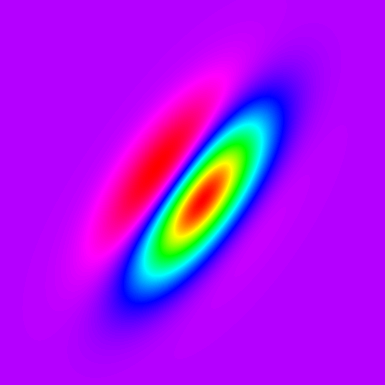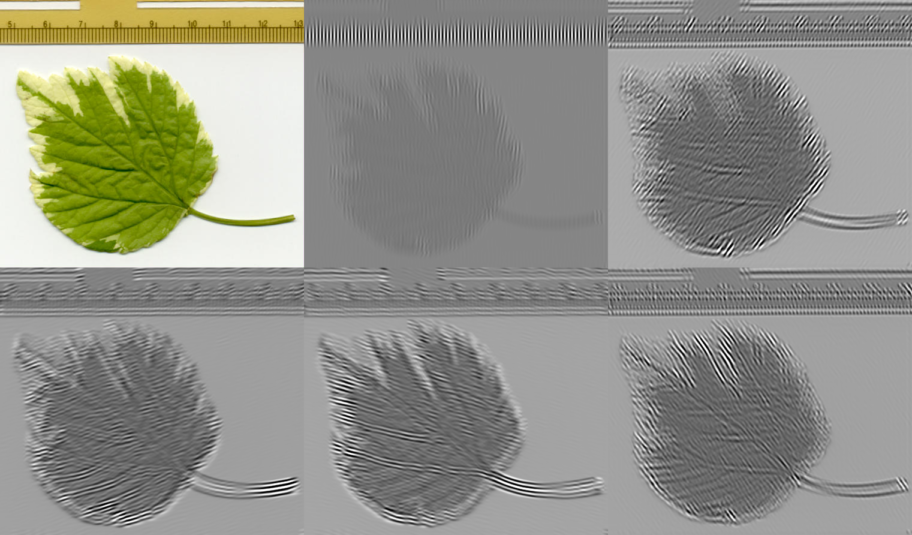# Gabor Filter scriptThe content of this page has not been vetted since shifting away from MediaWiki. If you’d like to help, check out the how to help guide!Example of a two-dimensional Gabor filter kernel (with a spectrum LUT).

This is an example of how to create Gabor filters in Fiji using Beanshell scripting. The script will create and apply a set of Gabor filters to the currently selected image.

Five different parameters can be adjusted:

• Sigma, which defines the size of the Gaussian envelope
• Psi, the phase offset
• Gamma, which is the spatial aspect ratio, and specifies the ellipticity of the support of the Gabor function.
• Fx, the frequency of the sinusoidal component
• nAngles, the number of filter orientations

As result, the script will display the set of filters, the filtered version of the original image for each orientation, and the projections (average, min, max, mean and variance) of the stack of filtered images.

## Example

This is an example of the script results using the Leaf sample image (FileOpen SamplesLeaf (36K)) and sigma = 8.0, gamma = 0.25, psi = 0.0, Fx = 3.0, nAngles = 5. <div class="thumbnail" >Demonstration of a Gabor filter applied to the Leaf sample image. Five orientations are shown on the right (0°, 36°, 72°, 108° and 144°). The original Leaf picture is shown on the upper-left corner. </div>

## Code

``````import ij.*;
import ij.process.*;
import ij.plugin.filter.*;
import ij.plugin.ContrastEnhancer;
import ij.plugin.ZProjector;

/**
* This script calculates a set of Gabor filters over the selected image.
*
* Parameters: sigma, gamma, psi, Fx, nAngles
*/

// Sigma defining the size of the Gaussian envelope
sigma = 8.0;
// Aspect ratio of the Gaussian curves
gamma = 0.25;
// Phase
psi = Math.PI / 4.0 * 0;
// Frequency of the sinusoidal component
Fx = 3.0;

// Number of diferent orientation angles to use
nAngles = 5;

// copy original image and transform it to 32 bit
originalImage = IJ.getImage();
originalImage = new ImagePlus(originalImage.getTitle(), originalImage.getProcessor().convertToFloat());
width = originalImage.getWidth();
height = originalImage.getHeight();

// Apply aspect ratio to the Gaussian curves
sigma_x = sigma;
sigma_y = sigma / gamma;

// Decide size of the filters based on the sigma
largerSigma = (sigma_x > sigma_y) ? (int) sigma_x : (int) sigma_y;
if(largerSigma < 1)
largerSigma = 1;

ip = originalImage.getProcessor().duplicate();

sigma_x2 = sigma_x * sigma_x;
sigma_y2 = sigma_y * sigma_y;

// Create set of filters

filterSizeX = 19; //6 * largerSigma + 1;
filterSizeY = 19; //6 * largerSigma + 1;

middleX = (int) Math.round(filterSizeX / 2);
middleY = (int) Math.round(filterSizeY / 2);

is = new ImageStack(width, height);
kernels = new ImageStack(filterSizeX, filterSizeY);

rotationAngle = Math.PI/(double)nAngles;
// Rotate kernel from 0 to 180 degrees
for (i=0; i<nAngles; i++)
{
theta = rotationAngle * i;
filter = new FloatProcessor(filterSizeX, filterSizeY);
for (int x=-middleX; x<=middleX; x++)
{
for (int y=-middleY; y<=middleY; y++)
{
xPrime = (double)x * Math.cos(theta) + (double)y * Math.sin(theta);
yPrime = (double)y * Math.cos(theta) - (double)x * Math.sin(theta);

a = 1.0 / ( 2.0 * Math.PI * sigma_x * sigma_y ) *
Math.exp(-0.5 * (xPrime*xPrime / sigma_x2 + yPrime*yPrime / sigma_y2) );
c = Math.cos( 2.0 * Math.PI * (Fx * xPrime) / filterSizeX + psi);

filter.setf(x+middleX, y+middleY, (float)(a*c) );
}
}
kernels.addSlice("kernel angle = " + theta, filter);
}

// Show kernels
ip_kernels = new ImagePlus("kernels", kernels);
ip_kernels.show();

// Apply kernels
for (i=0; i<nAngles; i++)
{
theta = rotationAngle * i;
c = new Convolver();

kernel = (float[]) kernels.getProcessor(i+1).getPixels();
ip = originalImage.getProcessor().duplicate();
c.convolveFloat(ip, kernel, filterSizeX, filterSizeY);

is.addSlice("gabor angle = " + i, ip);
}

// Normalize filtered stack
c = new ContrastEnhancer();
for(int i=1 ; i <= is.getSize(); i++)
{
c.stretchHistogram(is.getProcessor(i), 0.4);
}

projectStack = new ImagePlus("filtered stack",is);
IJ.run(projectStack, "Enhance Contrast", "saturated=0.4 normalize normalize_all");

resultStack = new ImageStack(width, height);

zp = new ZProjector(projectStack);
zp.setStopSlice(is.getSize());
for (int i=0;i<=5; i++)
{
zp.setMethod(i);
zp.doProjection();